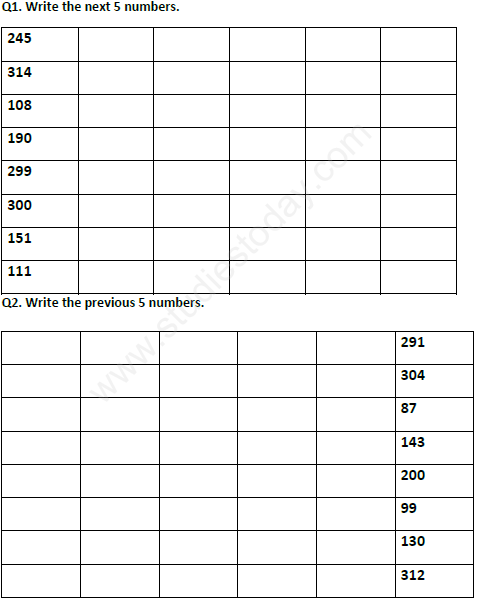# CBSE Class 2 Mathematics Revision Assignment Set C

Read and download free pdf of CBSE Class 2 Mathematics Revision Assignment Set C. Get printable school Assignments for Class 2 Mathematics. Class 2 students should practise questions and answers given here for All topics Mathematics in Class 2 which will help them to strengthen their understanding of all important topics. Students should also download free pdf of Printable Worksheets for Class 2 Mathematics prepared as per the latest books and syllabus issued by NCERT, CBSE, KVS and do problems daily to score better marks in tests and examinations

## Assignment for Class 2 Mathematics All topics

Class 2 Mathematics students should refer to the following printable assignment in Pdf for All topics in Class 2. This test paper with questions and answers for Class 2 Mathematics will be very useful for exams and help you to score good marks

### All topics Class 2 Mathematics AssignmentQ1. Write the multiplication facts for the following -:
a. 3 + 3+ 3+ 3+ 3+ 3 = ___________________
b. 5 + 5+ 5+ 5+5 = ___________________
c. 6+ 6+ 6 = ___________________

Q2. Write the division fact for the following-:
a. 6 x 4 = 24 ________________ ________________
b. 7 x 3 = 21 ________________ ________________
c. 9 x 2 = 18 ________________ ________________

Q3. Solve the following-:
a. 32/ 8= __________
b. 45 x 5 = __________
c. 60/10 = __________
d. 22 x 4 = __________

Q4. Solve the following word problems-:
a. It is Sonam’s birthday . she has given 2 toffees to 27 children. How many toffees has she distributed?
b. If 7 days make a week . How many weeks will be there in 63 days?

 CBSE Class 2 Mathematics Addition Assignment CBSE Class 2 Mathematics Before between and after Assignment CBSE Class 2 Mathematics Comparing Numbers Assignment CBSE Class 2 Mathematics Number Names Assignment CBSE Class 2 Mathematics Practice Assignment CBSE Class 2 Mathematics Revision Assignment Set A CBSE Class 2 Mathematics Revision Assignment Set B CBSE Class 2 Mathematics Revision Assignment Set C CBSE Class 2 Mathematics Revision Assignment Set D CBSE Class 2 Mathematics Revision Assignment Set E CBSE Class 2 Mathematics Revision Assignment Set F CBSE Class 2 Mathematics Story sums Assignment CBSE Class 2 Mathematics Subtraction Assignment

## More Study Material

Tags

### CBSE Class 2 Mathematics All topics Assignment

We hope you liked the above assignment for All topics which has been designed as per the latest syllabus for Class 2 Mathematics released by CBSE. Students of Class 2 should download and practice the above Assignments for Class 2 Mathematics regularly. We have provided all types of questions like MCQs, short answer questions, objective questions and long answer questions in the Class 2 Mathematics practice sheet in Pdf. All questions have been designed for Mathematics by looking into the pattern of problems asked in previous year examinations.

#### Assignment for Mathematics CBSE Class 2 All topics

Our team of expert teachers have referred to NCERT book for Class 2 Mathematics to design the Mathematics Class 2 Assignments. If you practice at least one test paper daily, you will get higher marks in Class 2 exams this year. Daily practice of Mathematics course notes and related study material will help you to clear all your doubts and have stronger understanding of all concepts. You can download all Revision notes for Class 2 Mathematics also from www.studiestoday.com absolutely free of cost.

#### All topics Assignment Mathematics CBSE Class 2

All questions and their answers for the assignment given above for Class 2 Mathematics have been developed as per the latest curriculum and books issued for the current academic year. The students of Class 2 can rest assured that the best teachers have designed the questions of Mathematics so that you are able to revise the entire syllabus if you do the assignments. Lot of MCQ questions for Class 2 Mathematics have also been given in the worksheets and assignments for regular use. All study material for Class 2 Mathematics students have been given on studiestoday.

#### All topics Assignment CBSE Class 2 Mathematics

Regular assignment practice helps to get a more comprehensive understanding of All topics concepts. Assignments play a crucial role in understanding All topics in CBSE Class 2. Students can download all the assignments of the same chapter in Class 2 Mathematics in Pdf format. You can print them or read them online on your computer or mobile.

#### CBSE Mathematics Class 2 All topics Assignment

CBSE Class 2 Mathematics latest books have been used for coming up with the latest questions and solutions for the above assignment. If you have revised all concepts relating to All topics then you should attempt all questions given in the test sheets above. We have also provided lot of Worksheets for Class 2 Mathematics which you can use to further make your self stronger in Mathematics

Where can I download in PDF assignments for CBSE Class 2 Mathematics All topics

You can download free Pdf assignments for CBSE Class 2 Mathematics All topics from StudiesToday.com

The assignments for All topics Class 2 Mathematics for have been made based on which syllabus

The All topics Class 2 Mathematics Assignments have been designed based on latest CBSE syllabus for Class 2 Mathematics issued for the current academic year

Can I download and print these printable assignments for Mathematics All topics Class 2

Yes, These printable assignments for All topics Class 2 Mathematics are free to download and print

How many topics are covered in All topics Mathematics assignments for Class 2

All topics given in All topics Mathematics Class 2 Book for the current academic year have been covered in the given assignment

Is there any charge for this assignment for All topics Mathematics Class 2